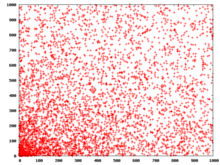# Pierpont prime

A Pierpont prime is a prime number of the form
2u3v + 1
for some nonnegative integers u and v. That is, they are the prime numbers p for which p  1 is 3-smooth. They are named after the mathematician James Pierpont.

It is possible to prove that if v = 0 and u > 0, then u must be a power of 2, making the prime a Fermat prime. If v is positive then u must also be positive, and the Pierpont prime is of the form 6k + 1 (because if u = 0 and v > 0 then 2u3v + 1 is an even number greater than 2 and therefore composite).

The first few Pierpont primes are:

2, 3, 5, 7, 13, 17, 19, 37, 73, 97, 109, 163, 193, 257, 433, 487, 577, 769, 1153, 1297, 1459, 2593, 2917, 3457, 3889, 10369, 12289, 17497, 18433, 39367, 52489, 65537, 139969, 147457, 209953, 331777, 472393, 629857, 746497, 786433, 839809, 995329, ... (sequence A005109 in the OEIS)

## Distribution of Pierpont primesDistribution of the exponents for the smaller Pierpont primes

Andrew Gleason conjectured there are infinitely many Pierpont primes. They are not particularly rare and there are few restrictions from algebraic factorisations, so there are no requirements like the Mersenne prime condition that the exponent must be prime. There are 36 Pierpont primes less than 106, 59 less than 109, 151 less than 1020, and 789 less than 10100; conjecturally there are O(log N) Pierpont primes smaller than N, as opposed to the conjectured O(log log N) Mersenne primes in that range.

## Pierpont primes found as factors of Fermat numbers

As part of the ongoing worldwide search for factors of Fermat numbers, some Pierpont primes have been announced as factors. The following table gives values of m, k, and n such thatThe left-hand side is a Pierpont prime when k is a power of 3; the right-hand side is a Fermat number.

m k n Year Discoverer
38 3 41 1903 Cullen, Cunningham & Western
63 9 67 1956 Robinson
207 3 209 1956 Robinson
452 27 455 1956 Robinson
9428 9 9431 1983 Keller
12185 81 12189 1993 Dubner
28281 81 28285 1996 Taura
157167 3 157169 1995 Young
213319 3 213321 1996 Young
303088 3 303093 1998 Young
382447 3 382449 1999 Cosgrave & Gallot
461076 9 461081 2003 Nohara, Jobling, Woltman & Gallot
672005 27 672007 2005 Cooper, Jobling, Woltman & Gallot
2145351 3 2145353 2003 Cosgrave, Jobling, Woltman & Gallot
2478782 3 2478785 2003 Cosgrave, Jobling, Woltman & Gallot

As of 2011, the largest known Pierpont prime is 3 × 27033641 + 1, whose primality was discovered by Michael Herder in 2011.

In the mathematics of paper folding, the Huzita axioms define six of the seven types of fold possible. It has been shown that these folds are sufficient to allow any regular polygon of N sides to be formed, as long as N > 3 and of the form 2m3nρ, where ρ is a product of distinct Pierpont primes. This is the same class of regular polygons as those that can be constructed with a compass, straightedge, and angle-trisector. Regular polygons which can be constructed with only compass and straightedge (constructible polygons) are the special case where n = 0 and ρ is a product of distinct Fermat primes, themselves a subset of Pierpont primes.

The smallest prime that is not a Pierpont (or Fermat) prime is 11; therefore, the hendecagon is the smallest regular polygon that cannot be constructed with compass, straightedge and angle trisector. All other regular n-gons with 3 ≤ n ≤ 21 can be constructed with compass, straightedge and trisector (if needed).

## Generalization

A Pierpont prime of the second kind is a prime number of the form 2u3v − 1, they are

2, 3, 5, 7, 11, 17, 23, 31, 47, 53, 71, 107, 127, 191, 383, 431, 647, 863, 971, 1151, 2591, 4373, 6143, 6911, 8191, 8747, 13121, 15551, 23327, 27647, 62207, 73727, 131071, 139967, 165887, 294911, 314927, 442367, 472391, 497663, 524287, 786431, 995327, ... (sequence A005105 in the OEIS)

A generalized Pierpont prime is a prime of the formwith k fixed primes {p1, p2, p3, ..., pk}, pi < pj for i < j. A generalized Pierpont prime of the second kind is a prime of the formwith k fixed primes {p1, p2, p3, ..., pk}, pi < pj for i < j. Since all primes greater than 2 are odd, in both kinds p1 must be 2. The sequences of such primes in OEIS are: